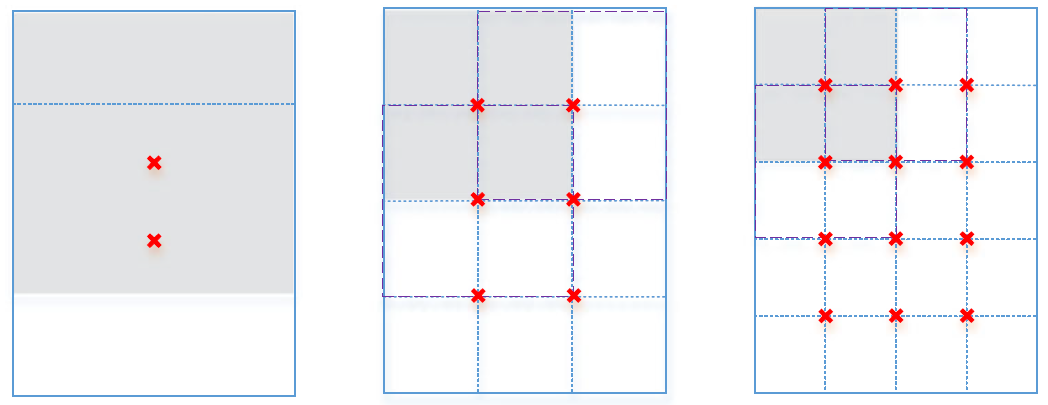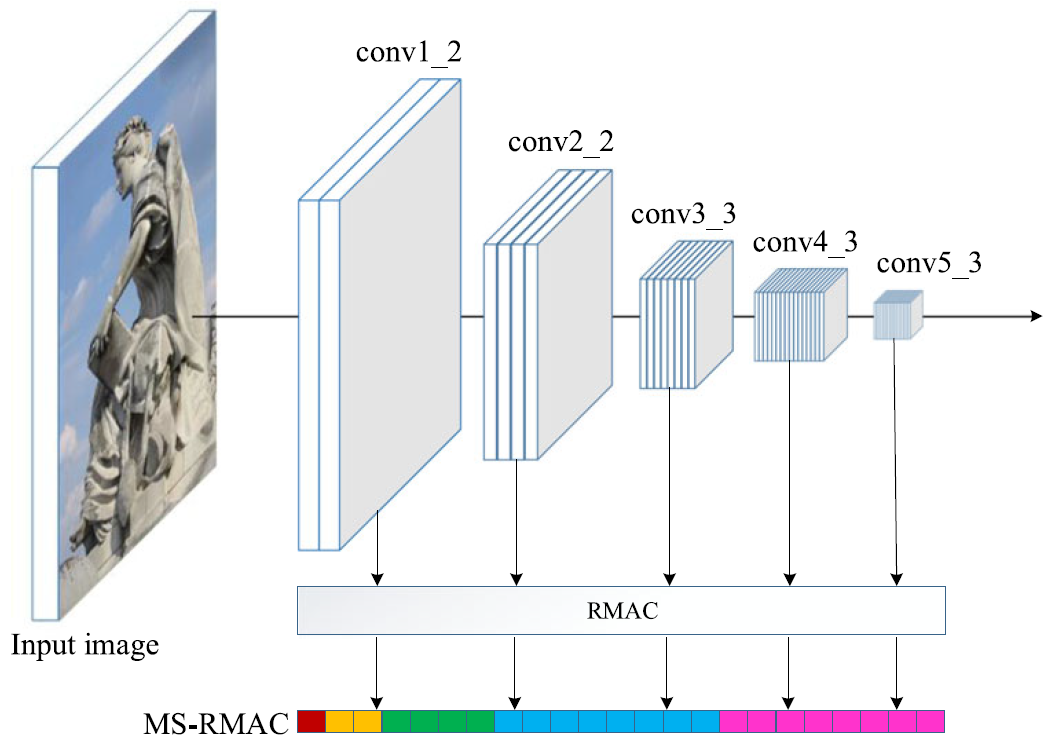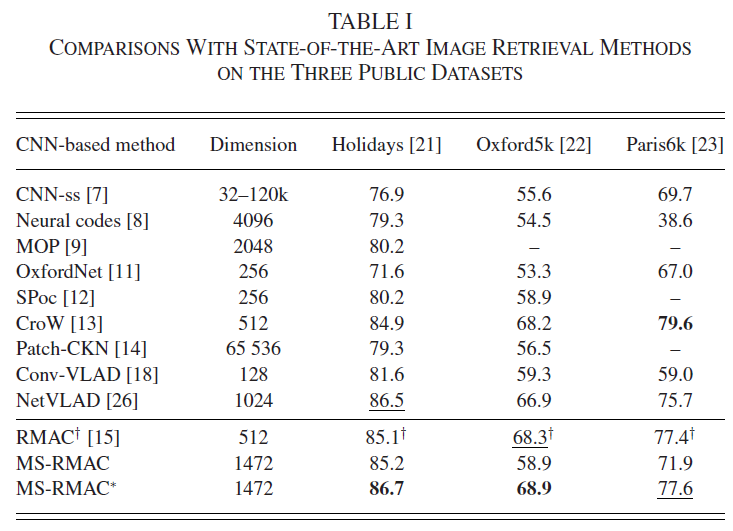“MS-RMAC: Multiscale Regional Maximum Activation of Convolutions for Image Retrieval” improves current Maximum Activation of Convolutions (MAC) feature for image retrieval by using multi-layer and regional MAC. It is published in IEEE Signal Processing Letters in 2017.

# Summary

• Problem Statement
• Features from a single convolutional layer are not robust enough for shape deformation, scale variation, and heavy occlusion.
• Proposed Solution
• Extract multiscale (MS) regional maximum activation of convolutions features from different layers of the convolutional neural networks.
• Propose aggregating MS features into a single vector by a parameter-free hedge method for image retrieval.
• Contribution
• MS-RMAC feature is aggregating from MS local convolutional feature maps, which does not require PCA-whitening or specialized fine-tuning on the test dataset and can mimic the ability of SIFT descriptors and CNN category-level features.
• Find that features from higher layers capture more semantic information of objects, and thus perform better than features from lower layers.
• Propose parameter-free weighting schemes that boost the effect of highly active semantic responses and improve retrieval accuracy.

# Methodology

## MS-RMAC RepresentationFigure 1: Sample regions extracted at three different scales (l = 1, …, 3). The top-left region of each scale (gray colored region) and its neighboring regions toward each direction (dashed borders) are highlighted. We depict the centers of all regions with a red cross.

### Computing RMAC

As shown in Fig. 1, we uniformly sample regions of width $2min(W,H)/(l+1)$ at each scale $l$. Also, the regions are sampled to allow around 40% overlap between consecutive retions. Finally, we sum all regions features into a single vector:

• Notation
• $j$: the layer number of convolutional feature maps
• $F_j$: RMAC features
• $N$: the total region number

## Computing MS-RMACFigure 2: Flowchart of the proposed MS-RMAC feature extraction method. Multiple RMAC features from different layers are concatenated into a vector.

Instead of using the final convolutional layer RMAC feature, we propose to use features extracted from multiple convolutional layers.

• Notation
• $MF$: proposed MS-RMAC feature
• $L$: the total layer number

The image search is then performed by finding the nearest database image to the query and sorting image based on the MS-RMAC feature Euclidean distance, formally

• Notation
• $d(q,p)$: distance between two images $p$ and $q$
• $\alpha_j$: weight of different convolutional feature maps, and $\sum^L_{j=1}\alpha_j=1$

## Hedge Weight for MS-RMAC

The standard parameter-free hedge algorithm is proposed to tackle decision-theoretic online learning problems in a multiexpert multiround setting. And it can be used to calculate the weights $\alpha_j$.

In round $t$, the hedge algorithm tries to calculate the weights $\alpha_t=(\alpha_{1,t}, \cdots, \alpha_{L,t})$. The loss of expert $j$ is computed as

• Notation
• $S_{j,t}$: the retrieval accuracy only using one layer RMAC feature
• $S_t$: the retrieval accuracy using all layer RMAC features with the weights in round $t$.

The standard parameter-free hedge algorithm generates a new weight distribution on all experts by introducing a regret measure defined by

where the weighted average loss among all experts is computed as $\overline{l}_{j,t}=\sum^L_{j=1}\alpha_{j,t}l_{j,t}$.

By minimizing the cumulative regret $R_{j,t}=\sum^t_{\tau=1}r_{j,\tau}$ in the first $t$ rounds to any expert $j$, the weights will be generated.

# ExperimentsThe reason why the proposed MS-RMAC feature performs well is two fold:

1. Visual representations using MS hierarchical RMAC features are more effective than single-scale CNN features.
• With CNN features from multiple scale, the proposed feature contains both category-level semantic information and fine-grained details information, which account for appearance changes caused by illumination variation, shape deformation, heavy occlusion, and background clutters.
2. The hedge weight method for MS-RMAC* is suitable for boosting the effect of highly active semantic responses and improves image retrieval accuracy.